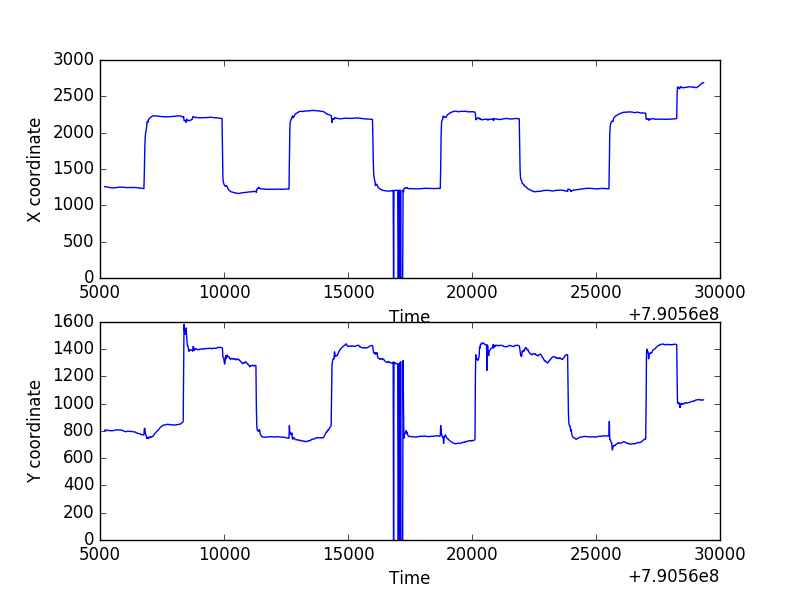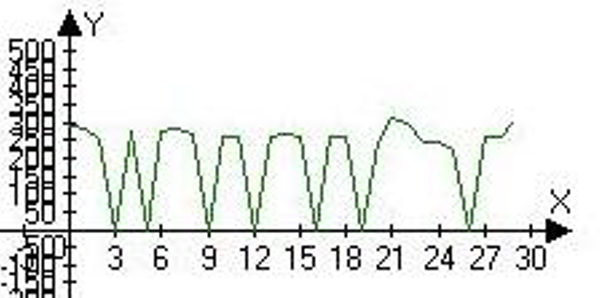Howdy, Stranger!

It looks like you're new here. If you want to get involved, click one of these buttons!

Supported by

Draw gaze-coordinates

Hi

I want to try eyeblink detection using eyetribe. I'm thinking of using gaze-coordinates to Identify blink (when

coordinates are zero). is this concept is ok ?

how can I plot eye gaze coordinates so that X-axis denotes the time whereas Y-axis denotes the gaze point
i.e. the point where the person is looking at.

Thanks

• I want to try eyeblink detection using eyetribe. I'm thinking of using gaze-coordinates to Identify blink (when coordinates are zero). is this concept is ok ?

In principle, yes. But be aware that 0 values simply indicate data loss, which can also occur for reasons other than blinks, such as failure to track the eye.

how can I plot eye gaze coordinates so that X-axis denotes the time whereas Y-axis denotes the gaze point i.e. the point where the person is looking at.

That is an extremely general question.What does your data look like, and what software do you want to use for plotting?There's much bigger issues in the world, I know. But I first have to take care of the world I know.
cogsci.nl/smathot

• My data is the output of eyetribe in .txt format , the experiment setting is to track eye gaze coordinates

and pupil size while the user is doing web based test cognitive test.

is there other reliable approach for eye blink ?

I think we should probably take a step back. Yes, there are, of course, other and better approaches to detect eye blinks than just looking at zeros. But I have the impression that you have very little experience working with this kind of data, and no idea at all how to write a script to parse it. Is that correct? If so, then this is going to be challenging!

I would start by defining clearly what you have done, what your data looks like, and what exactly you want to know. Then you can think of (and we can help you) what the easiest approach is.

For example, if you upload the datafile here, then I can show you a simple Python script that plots x, y and coordinates over time. That would be a start, and it's easy. (Although you would have to be able to run a standalone Python script.) But that's probably not your end goal, is it?

Cheers,
Sebastiaan

There's much bigger issues in the world, I know. But I first have to take care of the world I know.
cogsci.nl/smathot

• edited December 2016

HI sebastiaan

Yes , I have just started using eyetribe .

I want to find eyeblink rate , for example giving some task to the user and record eye data . After that detect eye blinks per minute ( for example ).

Also , I have another issue may be I can deal with it later , When I record data using eyetribe UI the file size becomes too large .

I suggested detect blinks from gaze coordinates because I have found a recent paper that used gaze

(x,y) to find blinks . They say that

( The Graphs are drawn based on the eyegaze coordinates that are collected from Eyetribe. Along the X-axis denotes the time whereas Y-axis denotes the gaze point i.e. the point where the person is looking at. The Eyetribe raw data's coordinate value is zero, indicating probably a blink. So, when the graph touches the X-axis it is a blink )

I have attached .csv for gaze coordinates that I downloaded fron eyetribe (eyeproof tool ). image from the paper for blinks and eyetribe sample .txt file .

Thanks for help

• Here's a simple script that parse the datafile and plots it as a figure, or rather two separate figures, one for the x coordinate and one for the y coordinate:

``````import json
from matplotlib import pyplot as plt

SRC = 'data.json'

# Read the datafile as text string
with open(SRC) as fd:
# Create empty lists to store the timestamp (t), and x and y coordinates
t = []
x = []
y = []
# Walk through each line of the datafile
for line in data.split('\n'):
try:
# Parse the line, which is JSON, into a dict
except:
print('Failed to decode', line)
continue
# Get t, x, and y from the dict and append them to the list
t.append(d['values']['frame']['time'])
sample = d['values']['frame']['avg']
x.append(sample['x'])
y.append(sample['y'])
# Plot the data!
plt.subplot(211)
plt.plot(t, x)
plt.xlabel('Time')
plt.ylabel('X coordinate')
plt.subplot(212)
plt.plot(t, y)
plt.xlabel('Time')
plt.ylabel('Y coordinate')
plt.savefig('data.png')
plt.show()
``````

For this particular datafile, it will give you something like this:But if you want to work with this data, you'll have to be a bit more independent than you are now! Do you understand what the script above does, and how you should run it? If not, then you can do one of the Python tutorials:

Once you have a basic grasp of what you're doing, we can help you. But we're not going to do the analysis for you!

Also , I have another issue may be I can deal with it later , When I record data using eyetribe UI the file size becomes too large .

Well, it's a lot of data!

There's much bigger issues in the world, I know. But I first have to take care of the world I know.
cogsci.nl/smathot

• Thanks ,

I will go through the code to understand how it works . which data should be used for this is it .csv or .txt

? . Also I have designed a web based task that I will use it to ask the users to do it while their eye being

tracked , after finishing the task is there is a way to import the data rather than using standard eyetribe

sdk ? because I think the data file will be in large size .

Regards

The file has to be in a .json file format if you want to use Sebastiaan's script directly. However, the main idea of the code should work for other file format as well. You might need to tweak a couple of things.

Eduard

• Thanks eduard , How can I convert my file to .json file format ?

• Hi,

• Here is the website of the `json` people. Read it to understand how that file format is structured.

• here is a file converter, that might be useful to you. It was literally the first hit on google when I looked for "JSON file format conversion". You could have done just the same.

Before, asking a question here, try first to fix your problem yourself. Google has most of the answers. If you can't find anything, you can ask here on the Forum of course, but this shouldn't be your first choice.

Eduard

• edited December 2016

Hi
I have run the code , but I have an issue .

1. I have recorded my eye movements then I imported the data from eyetribe UI , it take very long time to complete and the file size is large . Is there is a way to record the data that can give reasonable time and size ?
2. In the code provided does it reads eye gaze coordinates ? I need to draw X-axis as the time whereas Y-axis denotes the gaze points . How can I locate Gaze coordinates from the raw data file ?Thanks